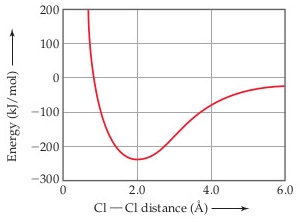# Problem: The following plot shows the potential energy of two Cl atoms as a function of the distance between them.What is the approximate bond strength for the Cl-Cl bond in Cl2 from this graph?

###### FREE Expert Solution

We’re asked to determine the approximate bond strength for the Cl-Cl bond in Cl2 based on the given graph showing a plot of the potential energy of two Cl atoms as a function of the distance between them.

Recall that the bond energy is the energy required to break a bond (pull two atoms completely apart)

• it is the same as the depth of the “well” in the potential energy curve.

The bond energy also determines the strength of the bond in a compound.

the higher the bond energy, the higher is the energy required to break up the molecule/compound into its individual atoms
higher bond energyhigher bond strength

This means that for the given plot, the bond energy can be measured at the point wherein the Cl atoms have the strongest attractive forces but not too close together that they don't repel each other.

98% (155 ratings)###### Problem Details

The following plot shows the potential energy of two Cl atoms as a function of the distance between them.What is the approximate bond strength for the Cl-Cl bond in Cl2 from this graph?